# 6 Simulating Data with R

(投影片 / 程式碼 / 影片)

## 6.1 分配 (Distribution)

• R 內建許多統計分配的函數，例如 *unif (均勻分配, Uniform), *norm (常態分配, Normal), *binom (二項分配, Binomial), *pois (泊松分配, Poisson) 等

• 每種分配都有一個「家族」的函數，用來做不同事情。例如，以常態分配為例 (詳見說明文件 ?dnorm)：

• dnorm(): 常態分配的機率密度
• pnorm(): 常態分配的累積機率密度
• rnorm(): 從常態分配抽取出來的樣本 (隨機數)
• qnorm(): 常態分配的 分位數 (quantile function)

### 6.1.1dnorm()

1. 製造一個 (間距很小的) 數列
2. 為每一個數列裡的值計算出相對應的機率密度

$Height \sim Normal(\mu = 160, \sigma = 5)$

library(ggplot2)
ggplot2::theme_set(ggplot2::theme_bw())

height <- seq(140, 180, by = 0.01)
density <- dnorm(x = height, mean = 160, sd = 5)

df <- data.frame(
height = height,
density = density
)

ggplot(df) +
geom_point(aes(x = height, y = density),
size = 0.1)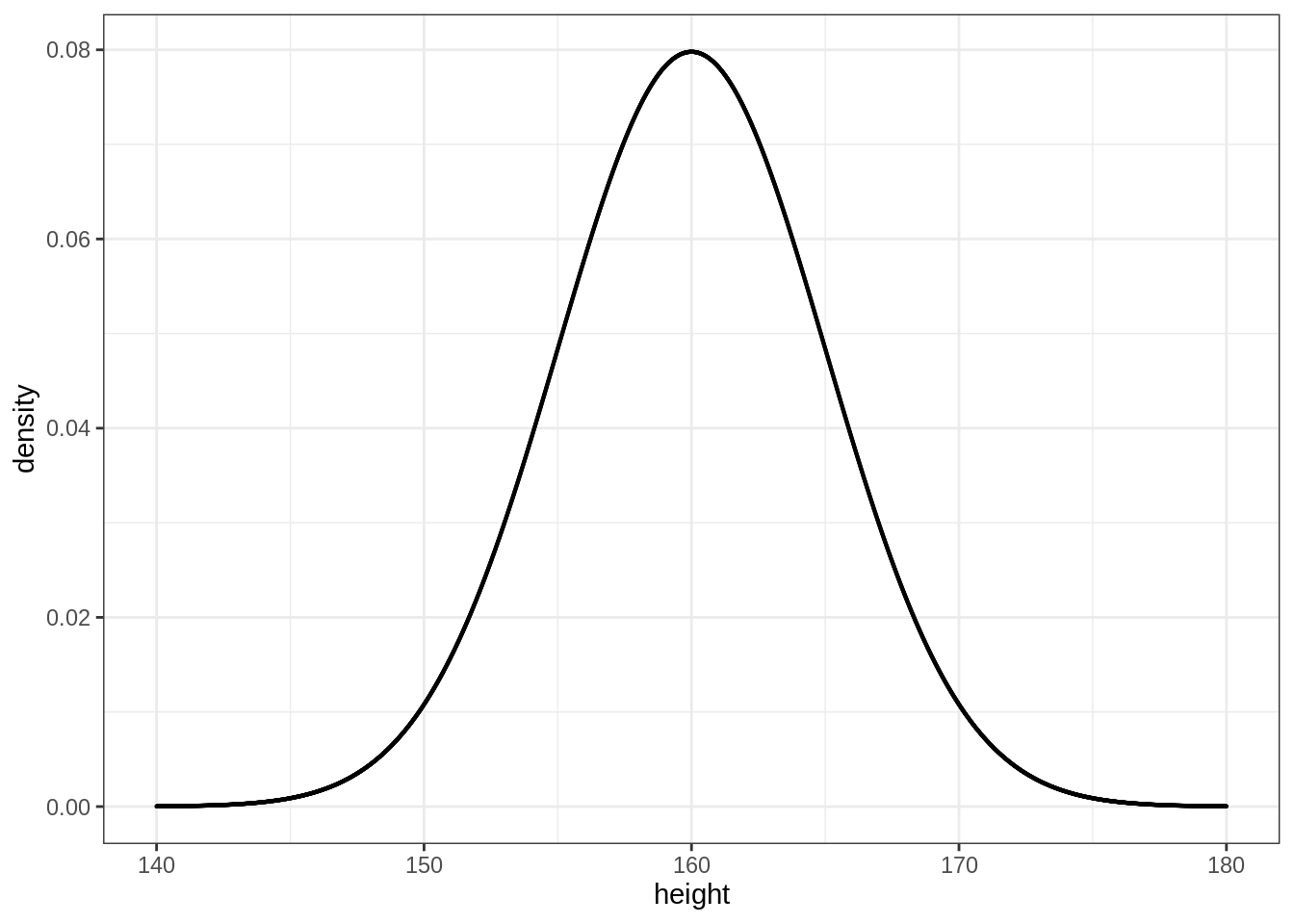### 6.1.2rnorm()

rnorm() 則可以讓我們從常態分配裡取抽取出樣本。例如，若我們想從剛剛的女性身高分配 ($$\mu = 160, \sigma = 5$$) 裡抽取出 3 個樣本：

rnorm(n = 3, mean = 160, sd = 5)
#>  153.7023 161.3947 160.6752

sampled_height <- rnorm(n = nrow(df), mean = 160, sd = 5)
mean(sampled_height)  # Sample's mean
sd(sampled_height)    # Sample's standard deviation
#>  159.9382
#>  5.010086

ggplot() +
geom_histogram(mapping = aes(x = sampled_height,
y = ..density..),
binwidth = 0.8, fill = "pink", color = "grey") +
geom_point(data = df,
mapping = aes(x = height, y = density),
size = 0.1)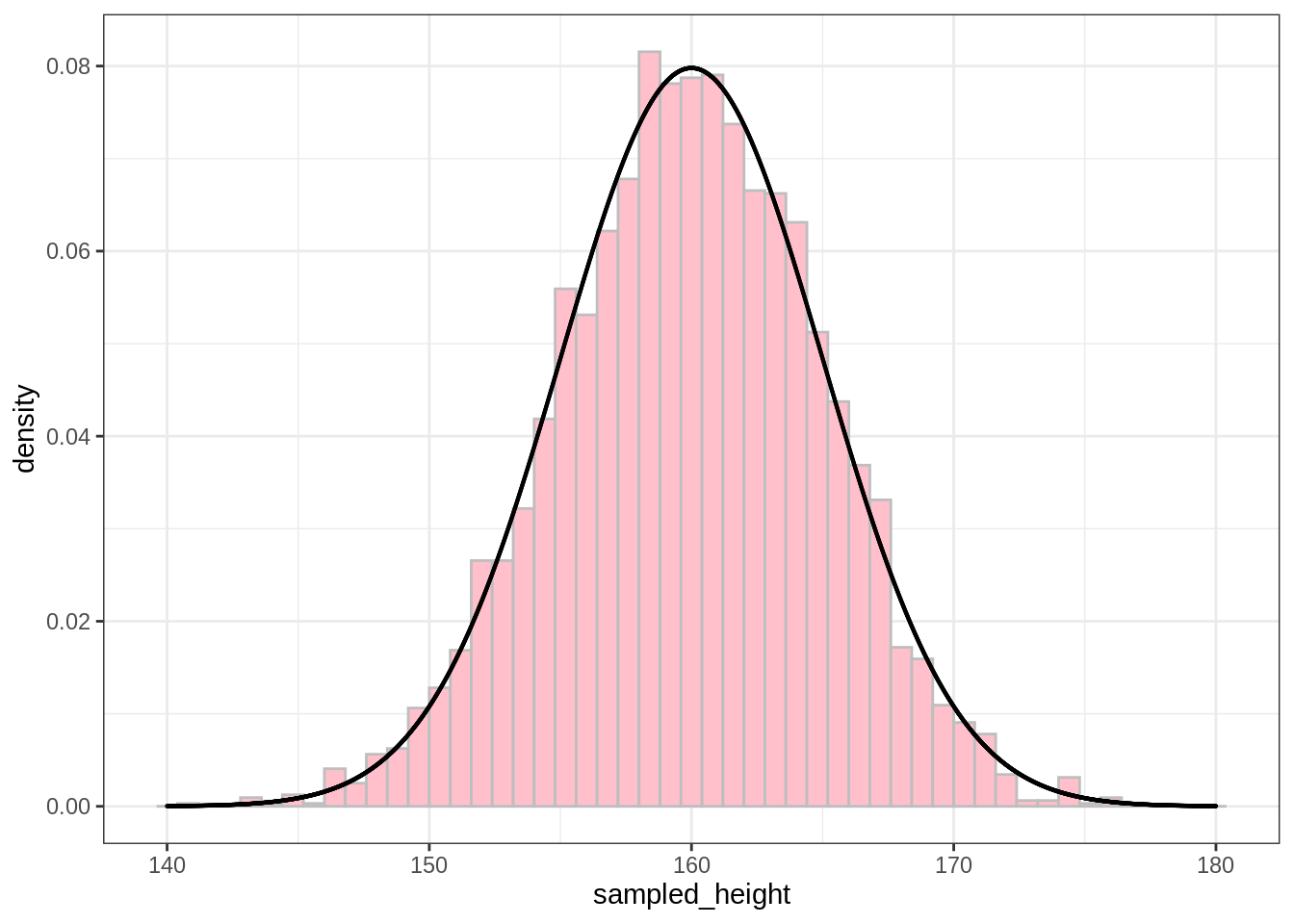## 6.2 相關 (Correlation)

# Perfect correlation
x <- 1:60
y <- x + 10
plot(x, y)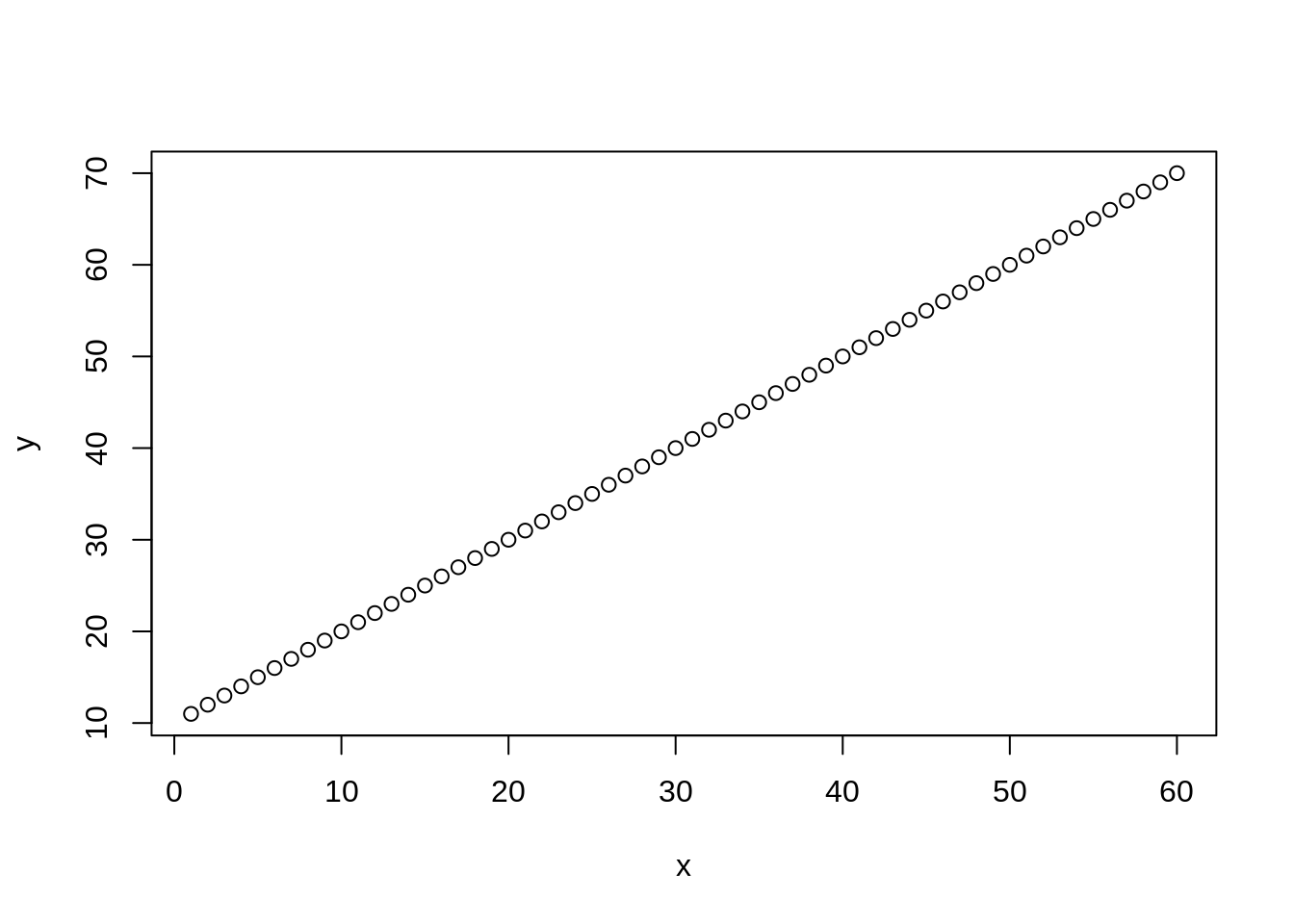cor(x, y)
#>  1

# Add some noise
y <- x + rnorm(n = length(x), mean = 0, sd = 7)
plot(x, y)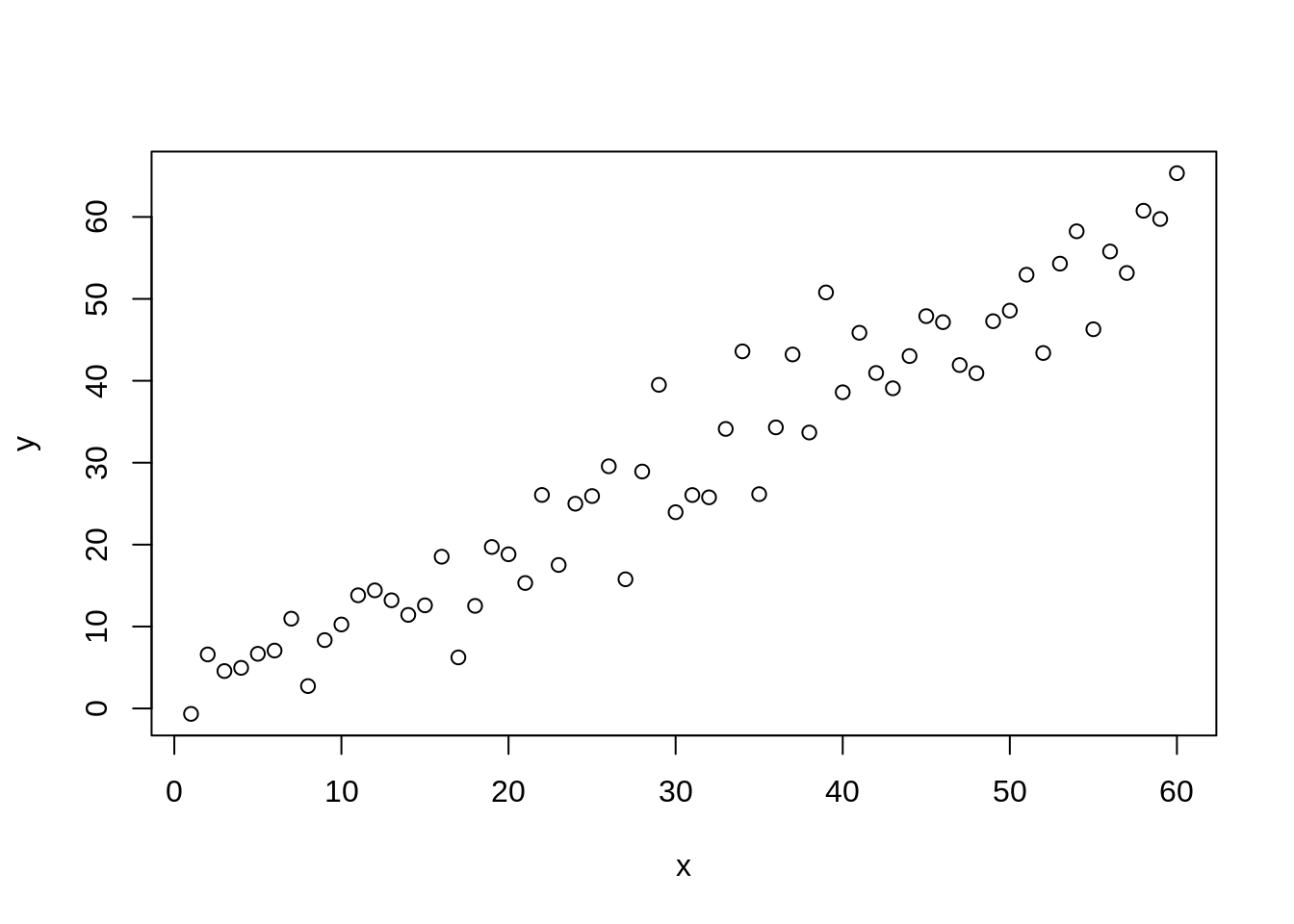cor(x, y)
#>  0.9624822

• 假設父親的身高 $$x_i \sim Normal(\mu=165, \sigma=5)$$
• 假設兒子的身高 ($$y_i$$) 受父親的直接影響：$$y_i \sim Normal(\mu = x_i, \sigma=5)$$
# Simulating correlation b/t two normal distributions
dad <- rnorm(n = 1000, mean = 165, sd = 5)
son <- rnorm(n = 1000, mean = dad, sd = 5)
plot(dad, son)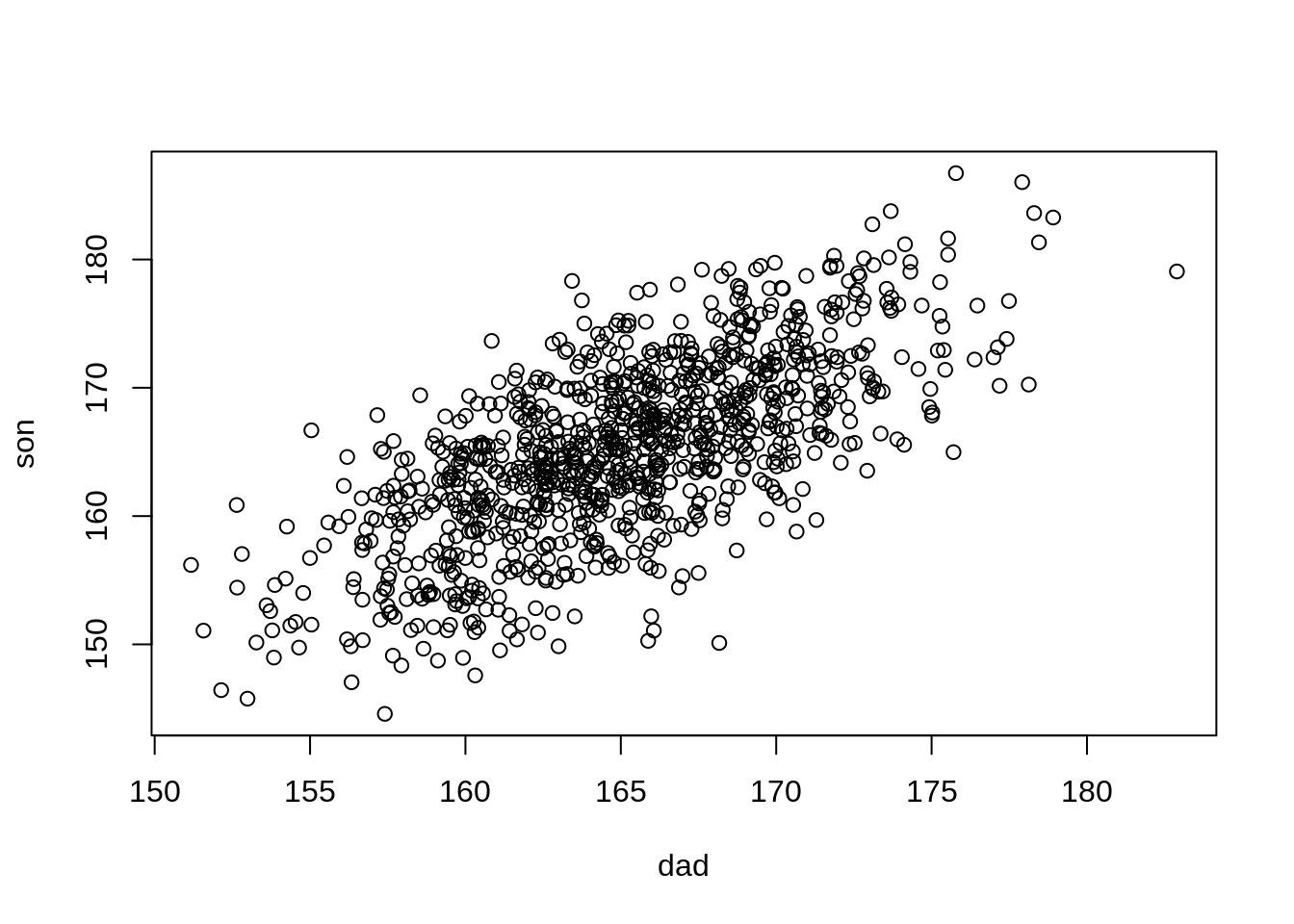cor(dad, son)
#>  0.6906082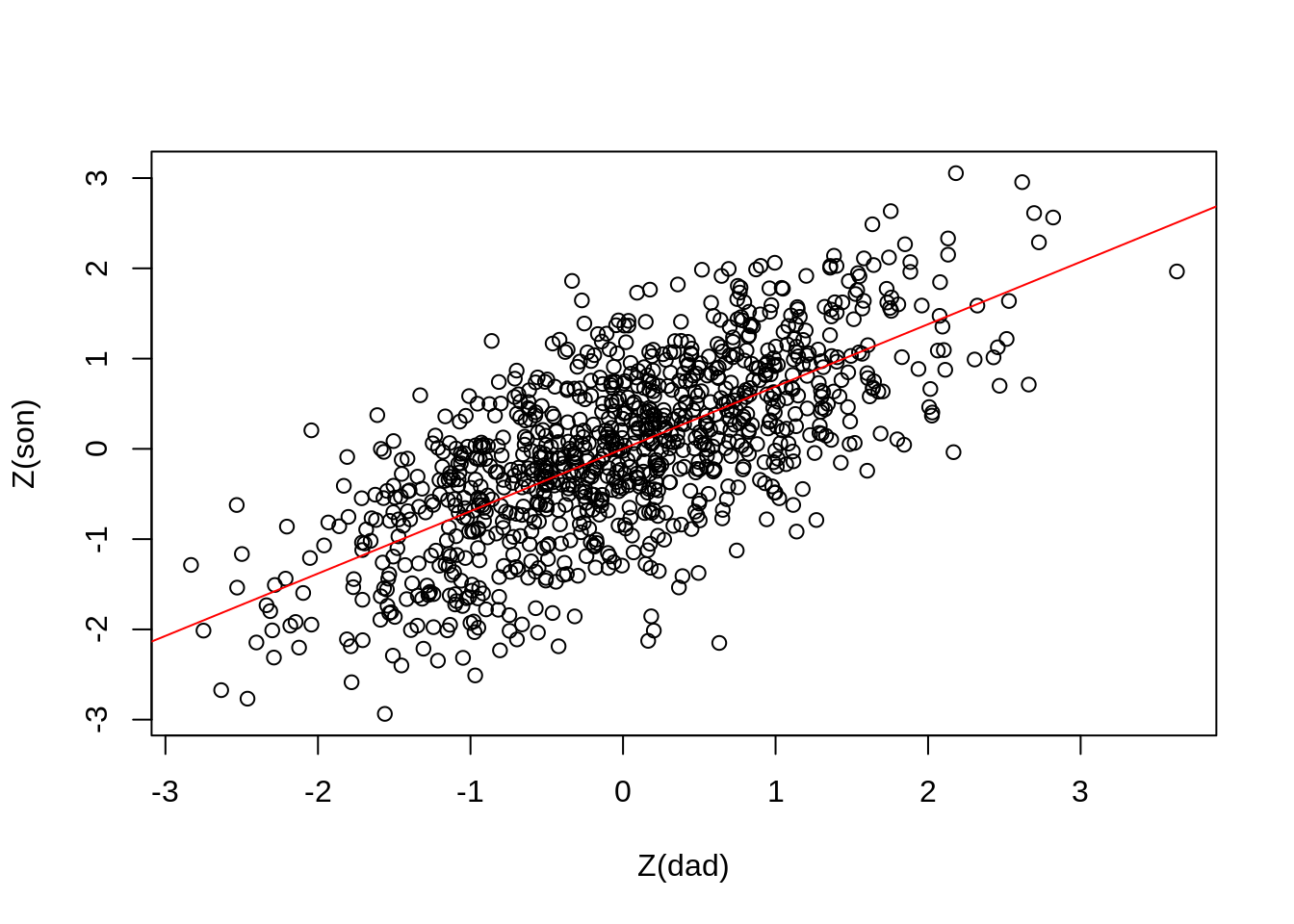# 標準化
Z <- function(x) (x - mean(x)) / sd(x)
son_std <- Z(son)

# Simple linear regression
summary(fit)
#>
#> Call:
#> lm(formula = son_std ~ dad_std)
#>
#> Residuals:
#>      Min       1Q   Median       3Q      Max
#> -2.58588 -0.48222  0.03348  0.51211  2.09151
#>
#> Coefficients:
#>               Estimate Std. Error t value Pr(>|t|)
#> (Intercept) -1.780e-15  2.288e-02    0.00        1
#> dad_std      6.906e-01  2.289e-02   30.17   <2e-16 ***
#> ---
#> Signif. codes:  0 '***' 0.001 '**' 0.01 '*' 0.05 '.' 0.1 ' ' 1
#>
#> Residual standard error: 0.7236 on 998 degrees of freedom
#> Multiple R-squared:  0.4769, Adjusted R-squared:  0.4764
#> F-statistic:   910 on 1 and 998 DF,  p-value: < 2.2e-16

## 6.4 混淆因子 (Confounder / Confounding Variable)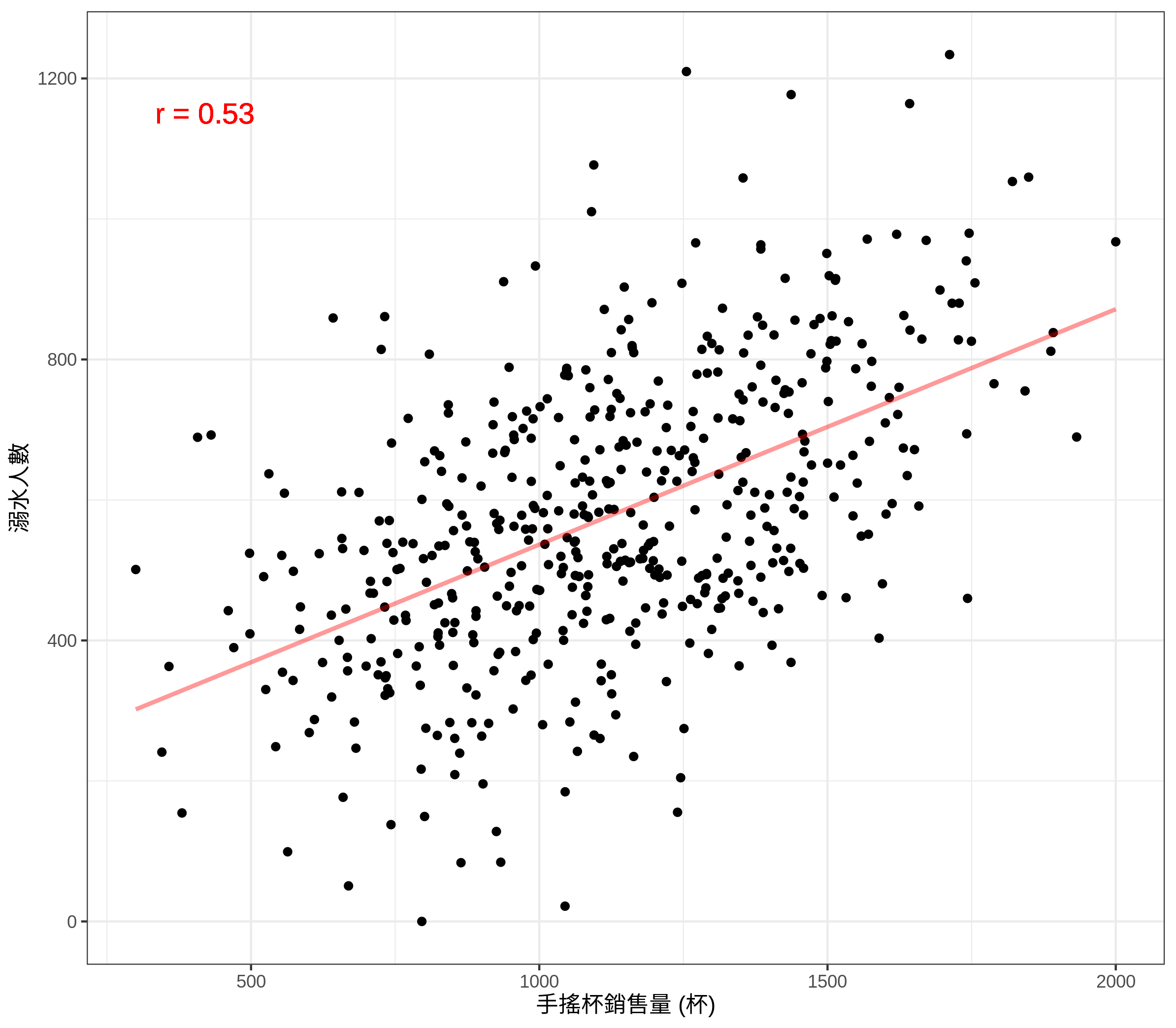Figure 6.1: 飲料銷售量與溺水人數關聯

• 本來獨立的獨變項與依變項，會因為混淆變項而產生關聯
• 本來關聯性不強的獨變項與依變項，會因為混淆變項而變得更強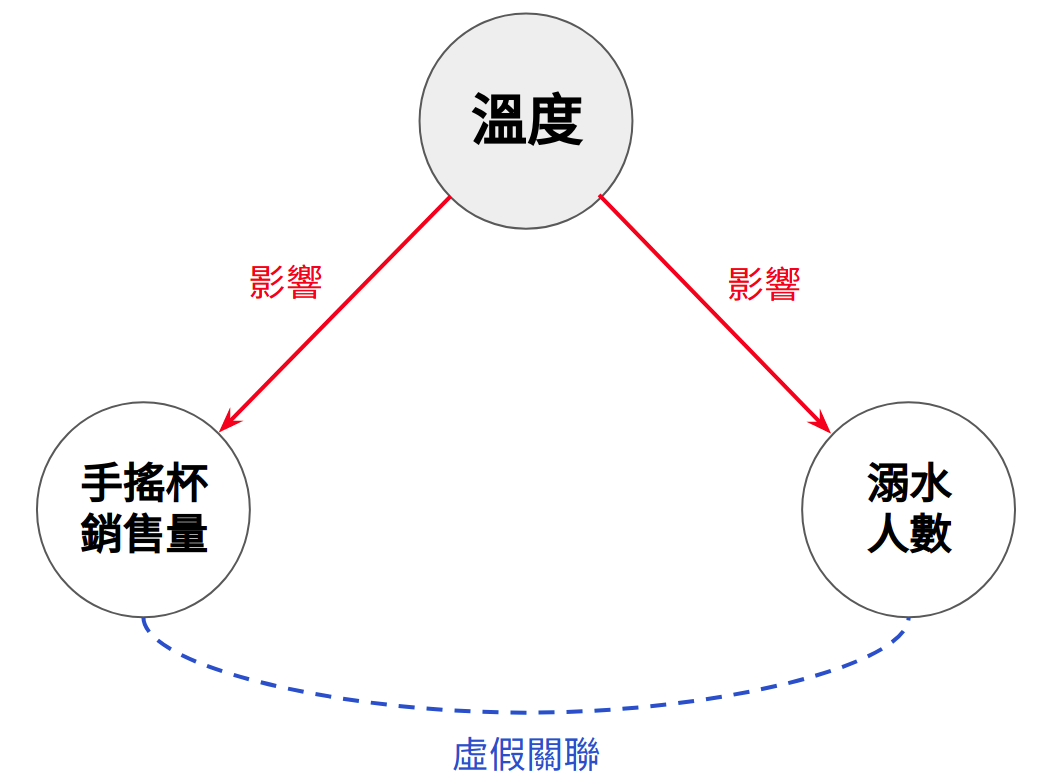Figure 6.2: 混淆因子 (Confounder)

### 6.4.1 Simulating Confounder

• temp: 溫度
• drown: 溺水人數。高溫會造成溺水人數增加。
• drink: 飲料銷售量。高溫會造成飲料銷售量增加。
set.seed(2021)  # Make results reproducible
N <- 500
temp <- rnorm(n = N, mean = 0, sd = 1)
drown <- rnorm(n = N, mean = temp, sd = 1)
drink <- rnorm(n = N, mean = temp, sd = 1)

• temp: 10-40 度
• drown: 0-1234 人
• drink: 300-2000 杯
source("functions.R")

# Scale to make data more realistic
df <- data.frame(
temp = minMaxScale(temp, m = 10, M = 40),
drown = minMaxScale(drown, m = 0, M = 1234),
drink = minMaxScale(drink, m = 300, M = 2000)
)

# Requires package psych, see functions.R
plotPairs(df)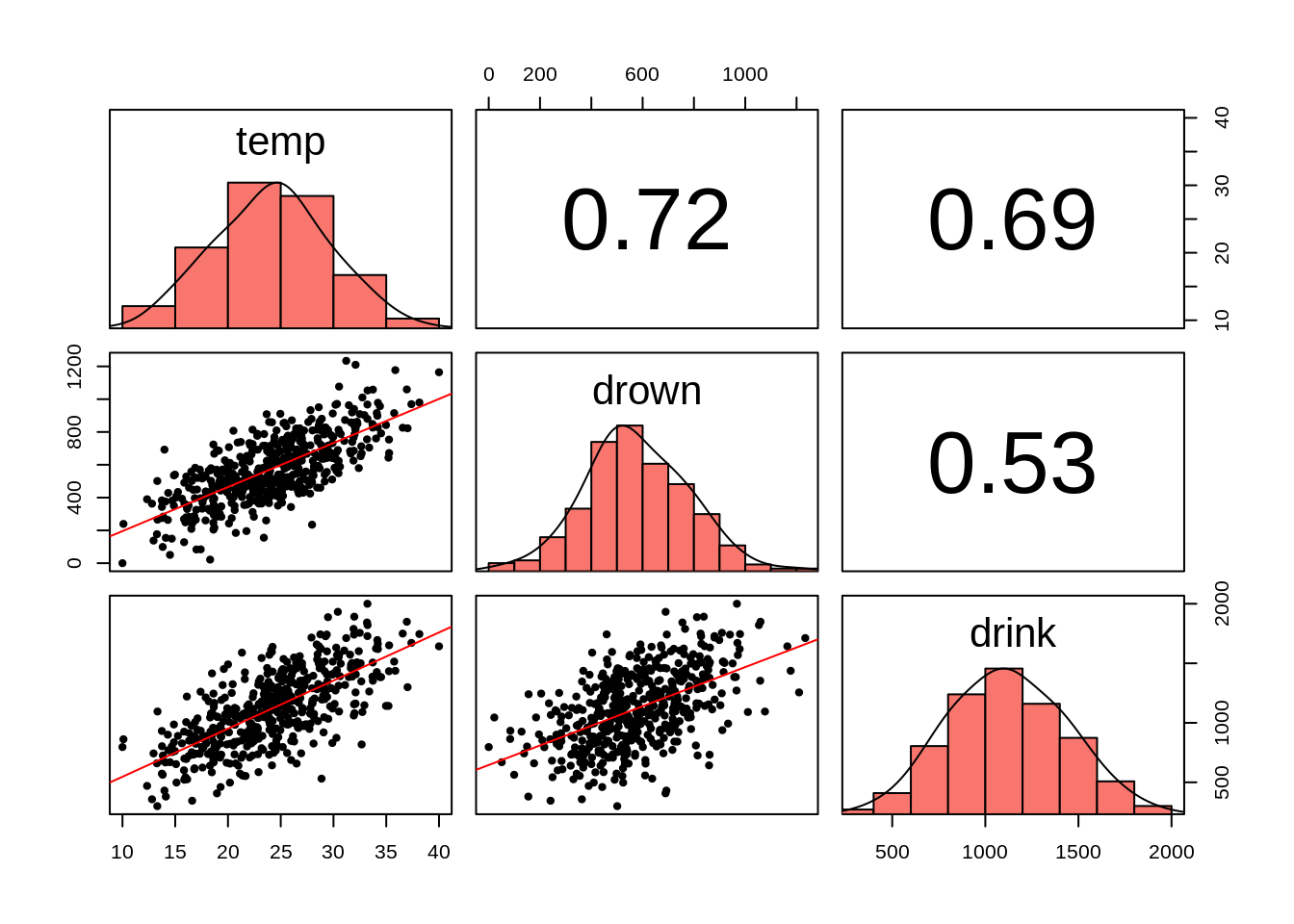### 6.4.2 Controlling for Confounder

1. 這些變項之間的因果結構 (這樣我們才知道混淆變項是誰)
2. 更複雜的統計模型用來控制混淆變項的影響

fit <- lm(Z(drown) ~ Z(drink), data = df)
summary(fit)$coefficients #> Estimate Std. Error t value Pr(>|t|) #> (Intercept) 1.896855e-16 0.03809807 4.978874e-15 1.000000e+00 #> Z(drink) 5.250932e-01 0.03813623 1.376888e+01 8.849357e-37 但若在迴歸模型中加入混淆變項，模型就會幫我們控制混淆變項的影響。這時，我們就可以得知在沒有混淆變項的影響下，手搖杯銷售量的提升對溺水人數的影響為何： fit <- lm(Z(drown) ~ Z(drink) + Z(temp), data = df) summary(fit)$coefficients
#>                 Estimate Std. Error      t value     Pr(>|t|)
#> (Intercept) 6.618100e-17 0.03092700 2.139910e-15 1.000000e+00
#> Z(drink)    4.512621e-02 0.04299789 1.049498e+00 2.944589e-01
#> Z(temp)     6.916087e-01 0.04299789 1.608471e+01 3.604313e-47

## 6.5 Don’t be a Control Freak

url <- 'https://rlads2021.github.io/lab/src/collider.csv'
head(df)
#> # A tibble: 6 x 3
#>   <dbl> <dbl> <dbl>
#> 1  158.  178.  173.
#> 2  161.  163.  164.
#> 3  162.  178.  175.
#> 4  151.  163.  157.
#> 5  170.  178.  178.
#> 6  173.  167.  172.

$Dad = \alpha + \beta_{Mom} Mom + \beta_{Son} Son$

fit <- lm(Z(dad) ~ Z(mom) + Z(son), data = df)
summary(fit)$coefficient #> Estimate Std. Error t value Pr(>|t|) #> (Intercept) 4.725574e-16 0.03186325 1.483080e-14 1.000000e+00 #> Z(mom) -5.193205e-01 0.03986636 -1.302654e+01 1.404517e-33 #> Z(son) 8.788082e-01 0.03986636 2.204386e+01 1.282788e-75 迴歸式的 $$\beta$$ 係數可能會讓我們得到一個結論： $$\beta_{mom}$$ = -0.519，所以…父親越高母親就越矮 所以這代表什麼？矮的女性喜歡跟高的男性結婚？矮的男性也喜歡跟高的女性結婚？要不然為何父親與母親的身高會是負向關係？ ## 6.6 對撞因子 (Collider) 其實在剛剛的資料裡，父親與母親的身高彼此是獨立的。它們之間的關聯之所以會產生，是因為在多元迴歸裡，我們控制了不該控制的變項。這筆身高的資料其實是來自於下圖的因果結構：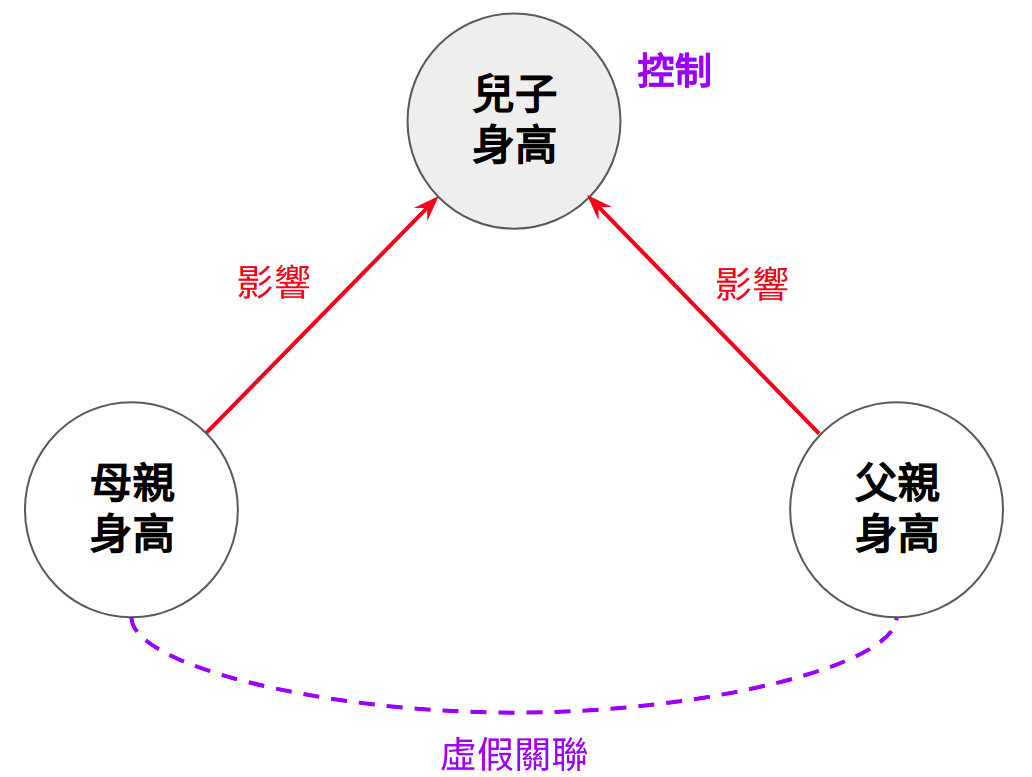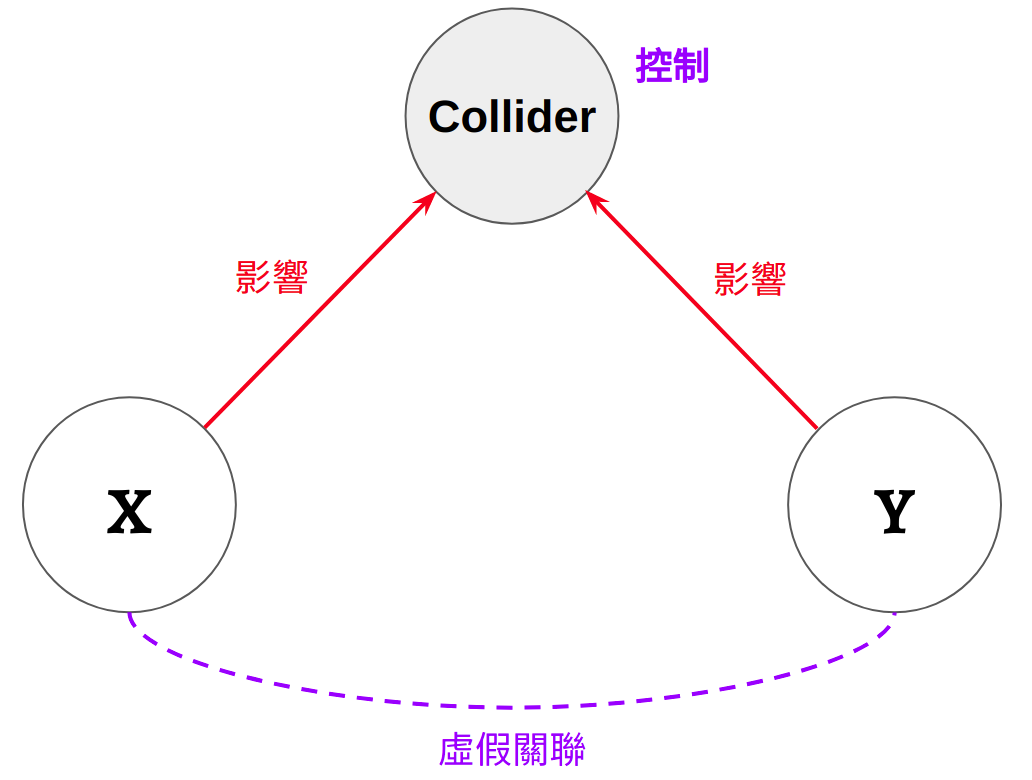Figure 6.3: 對撞因子 (Collider) 母親與父親的身高對兒子的身高皆有直接的因果影響。在這種情況下，如果我們在迴歸式裡面去控制兒子的身高，就會造成原本獨立的父、母親身高產生虛假關聯。在這種因果結構中，兒子身高的這個變項稱為對撞因子 (Collider)。對撞因子指的是被多個變項直接影響的變項。因為有多個因果影響「匯入」同一個變項，所以稱作對撞因子。 所以為何在控制了對撞因子之後，本來沒有關聯的變項會產生關聯呢？一個直觀的解釋是因為統計上的控制在概念上就是在固定某個變項的數值。所以在固定兒子的身高之後，一旦我知道母親的身高是矮的，我自然就會知道父親的身高是高的；反之亦成立，在固定兒子身高下，若父親是矮的，母親就會是高的20。接下來，我們來看看如何用 R 模擬這個現象。 ### 6.6.1 Simulating Collider 首先，我們透過 rnorm() 模擬出上方的因果結構： • mom: 母親身高 • dad: 父親身高 • son: 兒子身高。受父母身高的直接影響，且父親與母親的影響程度相同 set.seed(1914) # Make results reproducible N <- 500 # Sample size mom <- rnorm(N) dad <- rnorm(N) son <- rnorm(N, mom + dad) # dad & mom causally influence son's height # Scale variables to make them more realistic df <- data.frame( mom = 160 + 5 * mom, # Scale to Normal(160, 5) dad = 170 + 5 * dad, # Scale to Normal(170, 5) son = 170 + 5 * Z(son) # Scale to Normal(170, 5) ) 這裡，我們一樣將各變項進行線性轉換 (非必要)，讓資料看起來更直觀一點。我們將母親的身高平均轉換為 160；父親與兒子身高平均轉換為 170；三個變項的標準差皆轉換成 5。現在的這筆資料即與剛剛透過網路讀進來的 collider.csv 內容相同，我們可以透過相同的迴歸模型來檢驗看看： fit <- lm(Z(dad) ~ Z(mom) + Z(son), data = df) summary(fit)$coefficient
#>                  Estimate Std. Error       t value     Pr(>|t|)
#> (Intercept)  4.725574e-16 0.03186325  1.483080e-14 1.000000e+00
#> Z(mom)      -5.193205e-01 0.03986636 -1.302654e+01 1.404517e-33
#> Z(son)       8.788082e-01 0.03986636  2.204386e+01 1.282788e-75

fit <- lm(Z(dad) ~ Z(mom), data = df)
summary(fit)$coefficient #> Estimate Std. Error t value Pr(>|t|) #> (Intercept) -1.587427e-16 0.04476484 -3.546147e-15 1.0000000 #> Z(mom) 7.903449e-03 0.04480967 1.763782e-01 0.8600685 透過變項間的兩兩散布圖，我們也可以看到比較合理的趨勢：父母的身高是獨立的，兒子的身高與父母皆為正相關。 plotPairs(df)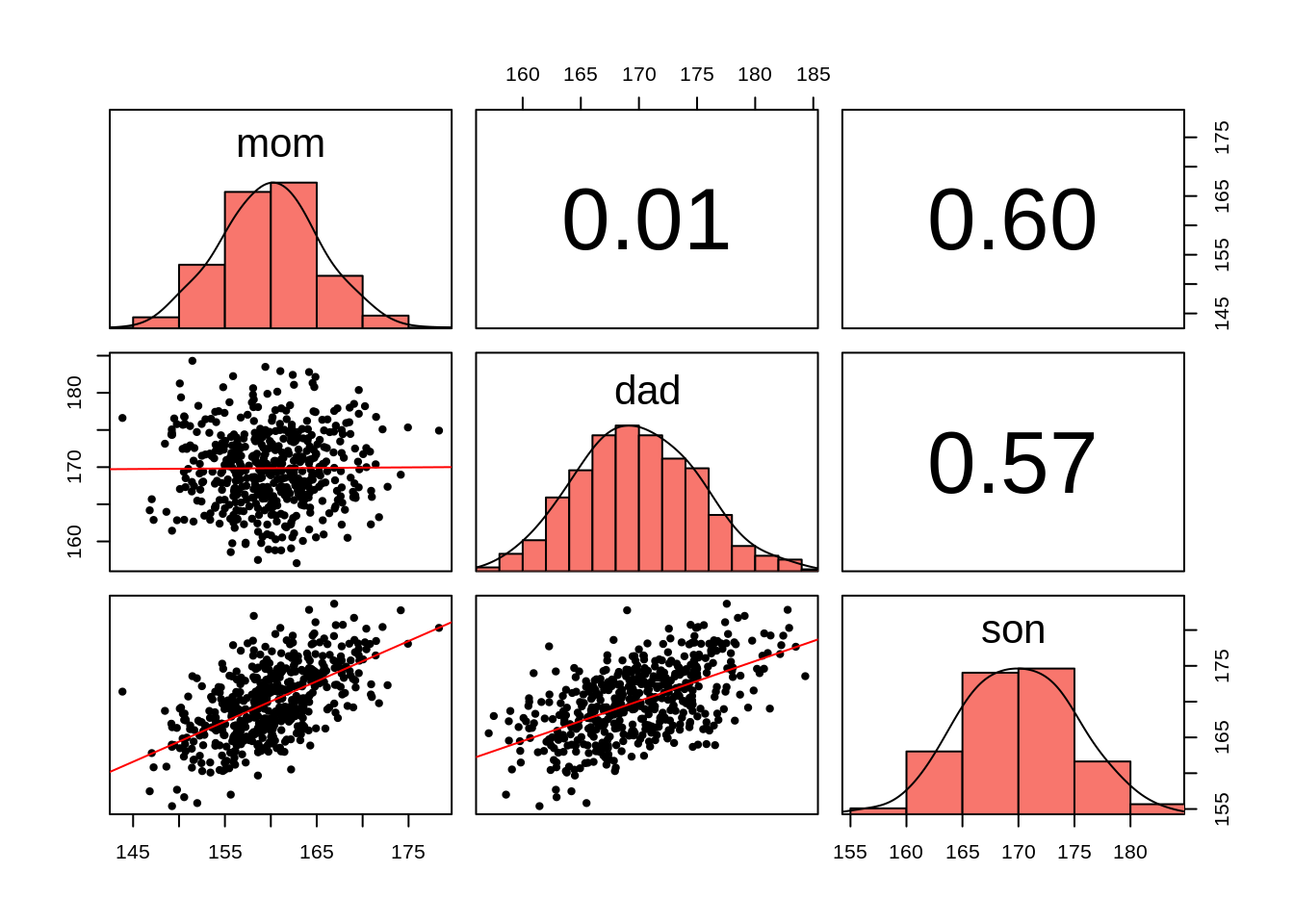### 6.6.2 Explaining Collider 前文只透過文字描述為何「控制」對撞因子會造成原本獨立的變項產生關聯。現在我們可以透過剛剛的身高資料來幫助我們理解這件事情。下方的這幾張散布圖中，灰色的點是所有的資料，黑色的點則是身高被固定在某個數值21的樣本，藍色的線則是透過這些黑色的點所算出來的迴歸線。在這裡我們可以看到控制兒子身高的作用：父親與母親的身高產生了負向相關。 plotRange <- function(df, lower, upper) { df_filt <- df[lower <= df$son & df$son < upper, ] r <- round(cor(df_filt$mom, df_filt\$dad), 3)

pl <- ggplot(mapping = aes(mom, dad)) +
geom_point(data = df, color = "grey", size = 1) +
geom_point(data = df_filt, size = 1) +
geom_smooth(data = df_filt, size = 1,
method = "lm", se=F) +
labs(subtitle = paste0("r = ", r, "; son's height fixed at ", lower, "~", upper, " cm"))
return(pl)
}
rng <- seq(165, 175, by = 1.5)
plts <- vector("list", length = length(rng) - 1)
for (i in seq_along(rng)) {
if (i == length(rng)) break
plts[[i]] <- plotRange(df, rng[i], rng[i + 1])
}
patchwork::wrap_plots(plts, ncol = 2)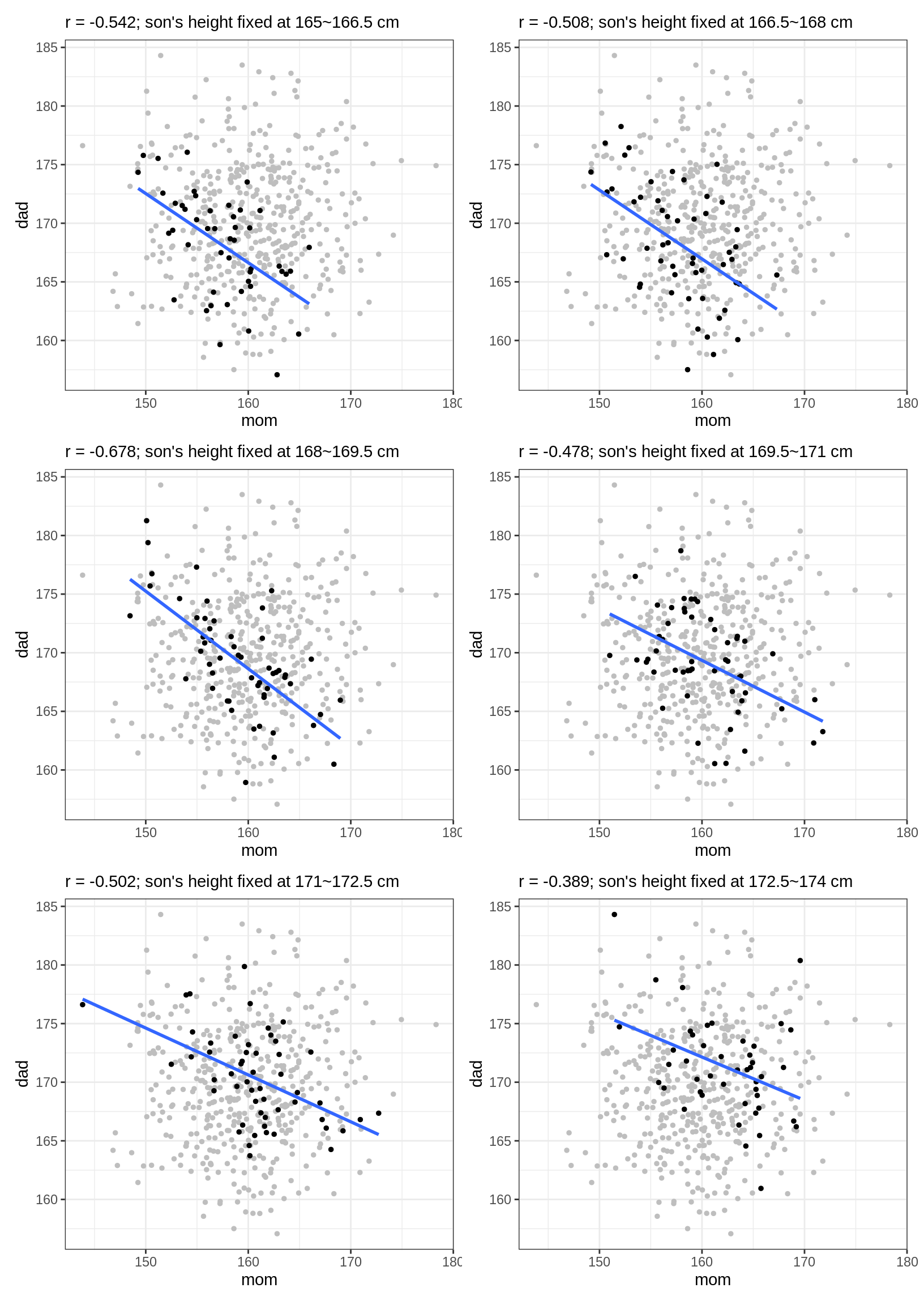## 進階閱讀

1. Pearl, J., Glymour, M., & Jewell, N. P. (2016). Causal inference in statistics: A primer
2. Pearl, J. (2009). Causality (2nd ed.)
3. McElreath, R. (2020). Statistical rethinking: A Bayesian course with examples in R and Stan (2nd ed.)